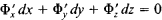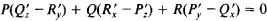# Pfaffian Equation

The following article is from The Great Soviet Encyclopedia (1979). It might be outdated or ideologically biased.

## Pfaffian Equation

an equation of the form

(1) X1 dx1 + X2dx2 + … + Xndxn = 0

where X1, X2, …, Xn are given functions of the independent variables x1, x2, …, xn. Such equations were studied by J. F. Pfaff in l814 and l815.

The solution of equation (1) is given by equations

f1(x1, x2,…, xn) = 0

(2) f2(x1, x2,…, xn) = 0

…………………

fm(x1, x2,…, xn) = 0

such that equation (1) is a consequence of these equations and of the equations df1 = 0, df2 = 0, …, dfm = 0. Equations (2) define the integral manifold of the Pfaffian equation (1). If an (n - 1)-dimensional integral hypersurface passes through every point of the n-dimensional space x1, x2,…, xn —that is, if equation (1) is integrated in terms of a single relation containing a single arbitrary constant, equation (1) is said to be completely integrable.

In the case of three independent variables x, y, and z, the Pfaffian equation can be written in the form

(1’) P dx + Q dy + R dz = 0

where P = P(x, y, z), Q = Q(x, y, z), and R = R(x, y, z). Geometrically, to solve equation (1’) is to find curves in the space x, y, z that are orthogonal at each of their points to the vector field P, Q, R— that is, curves whose normal plane at each point contains a vector of the field. Such curves are the integral curves of equation (1’). If a single equation Φ(x, y, z) = 0 is arbitrarily specified—that is, if integral curves are sought on an arbitrary smooth surface—then we can use equation (1’) and the equationto find, for example, dy/dx and dz/dx as functions of x, y, and z, and the problem reduces to integration of a system of two ordinary first-order differential equations. If this system is solved, a two-parameter family of curves is found, from which we determine the one-parameter family of integral curves of equation (1″) on the given surface Φ(x, y, z) = 0. This family of integral curves can be regarded as the intersection of the given surface and the one-parameter family of surfaces Φ1(x, y, z, c) = 0. In other words, the general solution of the Pffaffian equation (1’) consists of the two relations Φ(x, y, z) = 0 and Φ1(x, y, z, c) = 0, the first of which is arbitrary and the second of which is determined by the first. The Pfaffian equation (1’) is integrated in terms of a single relation F(x, y, z, c) = 0—that is, it is completely integrable—if the integrability conditionis satisfied identically in x, y, and z. Geometrically, this means that there exists a one-parameter family of integral surfaces of the Pfaffian equation (1ʹ) that are orthogonal at every point to the vector field (P, Q, R). Any curve on an integral surface is an integral curve of the Pfaffian equation (1″).

The theory of Pfaffian equations has been generalized to the case of systems of such equations, which play an especially important role in applications. Pfaffian equations and systems of such equations are encountered in the mechanics of nonholonomic systems (since nonholonomic constraints are Pfaffian equations connecting the virtual displacements) and in thermodynamics.

### REFERENCES

Rashevskii, P. K. Geomelricheskaia teoriia uravnenii s chastnymi proizvodnymi. Moscow-Leningrad, 1947.
Stepanov, V. V. Kurs differentsial’nykh uravnenii, 8th ed. Moscow, 1959.
Goursat, E. Leçons sur le problème de Pfaff. Paris, 1922.